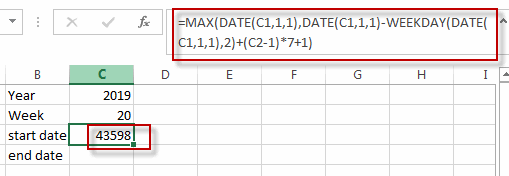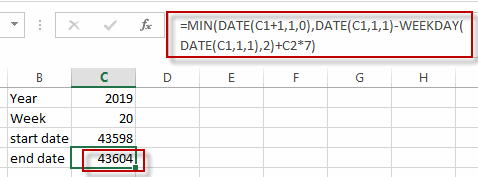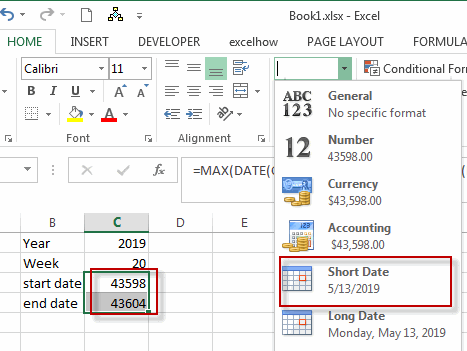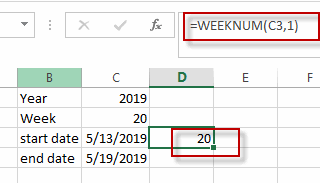# Converting Week Number to Date

This post will guide you how to convert a week number to a date with a formula in Excel. How do I calculate a date from a week number and a year with formula in Excel. Or how to get week number from a given date with formula in Excel.

## Convert Week Number to Date

Assuming that you have a week number and a year number in your worksheet, and you want to get the start date and end date in that give week in that year. How to achieve it.

You need to create a formula based on the MAX function, the DATE function and the WEEKDAY function to convert week number to a date in Excel. Just do the following steps:

#1 Type the year number in Cell C1, and type the week number in Cell C2.

#2 Type this formula into the formula box of the Cell C3, then press Enter key in your keyboard. The Serial number of the start date is calculated in Cell C3.

=MAX(DATE(C1,1,1),DATE(C1,1,1)-WEEKDAY(DATE(C1,1,1),2)+(C2-1)*7+1)#3 Type the following formula into the formula box of the Cell C4, and then press Enter key in your keyboard. And the serial number of the end date is calculated in Cell C4.

=MIN(DATE(C1+1,1,0),DATE(C1,1,1)-WEEKDAY(DATE(C1,1,1),2)+C2*7)#4 select the cells C3:C4 and then go to HOME tab, click Number format list box, and select Short Date item. The serial number are changed to a standard date format.  Or you can also select Long Date format from the drop-down list of the Number format.## Get Week Number From a Date

If you want to get the week number from a given date, then you can use the WEEKNUM function to quickly achieve it. Just type the formula into the Cell D3:

`=WEEKNUM(C3,1)`### Related Functions

• Excel MIN function
The Excel MIN function returns the smallest numeric value from the numbers that you provided. Or returns the smallest value in the array.The MIN function is a build-in function in Microsoft Excel and it is categorized as a Statistical Function.The syntax of the MIN function is as below:= MIN(num1,[num2,…numn])….
• Excel MAX function
The Excel MAX function returns the largest numeric value from the numbers that you provided. Or returns the largest value in the array.= MAX(num1,[num2,…numn])…
• Excel DATE function
The Excel DATE function returns the serial number for a date.The syntax of the DATE function is as below:= DATE (year, month, day)…
• Excel WEEKDAY function
The Excel WEEKDAY function returns a integer value representing the day fo the week for a given Excel date and the value is range from 1 to 7.The syntax of the WEEKDAY function is as below:=WEEKDAY (serial_number,[return_type])…
• Excel WEEKNUM function
The Excel WEEKNUM function returns the week number of a specific date, and the returned value is ranging from 1 to 53.The syntax of the WEEKNUM function is as below:=WEEKNUM (serial_number,[return_type])…